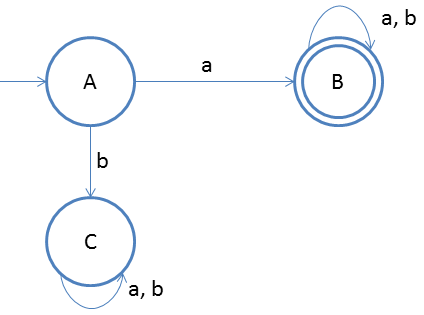# DFA String Examples

We will now discuss about string patterns such as, starting with some symbol, ending with some symbol, etc.

Design a DFA in which set of all strings can be accepted which start with a.
Given: Input alphabet, Σ={a, b}
Language L = {a, ab, aba, aabb, aaabbba, …..}

Clearly the language is infinite because there is infinite number of strings.The idea behind this approach is very simple, follow the steps below and you will understand.

1. We know that first input must be a and from start state on a we should go to final state, so two states are confirmed: start state and final state.
2. And if the first input is something else than ‘a’ then string should not be accepted. And we don’t care whatever comes on state C as state C now will work as dead state.
3. Once we reach final state we accept everything that is why we have a loop

### Testing

1. Lets take one input string abab
2. First input is a, so from state A we will go to state B
3. Second input is b, so from state B we will go to state B itself
4. Third input is a, so from state B we will go to state B itself
5. Fourth input is b, so from state B we will go to state B itself(final state)
After end of the string we are at final state so string is accepted.